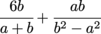# TSI Math: Simplifying Fractions Using the Least Common Denominator

What is the simplified form of the expression?

 Intermediate Algebra and Functions Rational and Exponential Expressions, Equations, and Functions Mathematics and Statistics Assessment Rational and Exponential Expressions, Equations, and Functions Product Type TSI TSI Mathematics and Statistics AssessmentTSI MathTSI Mathematics TSI Math Intermediate Algebra and Functions TSI Mathematics Intermediate Algebra and Functions Test Prep TSI

### Transcript

00:22

denominator Right Fractions with different denominators are like vinegar and

00:26

baking soda You can't add them without making a real

00:29

mess Yeah we've been there were tempted to go mad

00:31

scientist to here on you but we shouldn't Since it's

00:34

a difference of squares b squared minus a square there

00:37

Well it can be factored Right factor is b minus

00:40

a times b plus eh And a plus b equals

00:43

b plus eh Is a factor of b squared minus

00:46

a square Just multiply the first fraction sixty over a

00:49

plus b by being kind to say over b money

00:51

say which is one as long as b minus a

00:54

isn't zero to give a denominator of b squared minus

00:57

a squared See how that works That's what it looks

00:59

like All right Well now we add the fractions Six

01:02

b squared minus a b over b squared minus a

01:04

squared plus a bee And that gets us this thing

01:08

Six b squared minus a b plus eh b over

01:11

b squared minus squared Well the two a b terms

01:13

cancel out so we're left With the some six b

01:16

squared over b squared minus a squared that's it uh

01:19

give us the word problem is that it kind of 00:01:20.62 --> [endTime] funny Can't we go back to those